# Kerala Syllabus 7th Standard Maths Solutions Chapter 10 Money Math

## Kerala State Syllabus 7th Standard Maths Solutions Chapter 10 Money Math

### Money Math Text Book Questions and Answers

Math and commerce Textbook Page No. 130

Various commercial activities started very early in human history. At first it was just exchange of goods, such as two goats for one cow or five mangoes for a jackfruit.

At a later stage, different forms of currency was used to denote the value of goods, instead of the actual goods themselves. Much computation with numbers was necessary to keep such momentary transactions exact. Thus such computations also became a part of mathematics education.

Quintal, ton, tonne

In the past, different units were used in different parts of the world to measure length, weight and so on. Now these have been unified, with most countries using the metric system.

A unit of weight called quintal was in use in many countries during the olden days, denoting hundred times the basic unit of weight. Once the metric system was adopted, a quintal was fixed as 100 kilograms.

In the past, a ton in England meant 2240 pounds (1016 kilograms in the current system). In the metric system, 1000 kilograms is called a tonne. To distinguish between the two, a tonne is also known as a metric ton.

According to the common prefixes used in the metric system, a tonne, is a mega gram (1000000 grams).Chains of Commerce

In today’s world, there are numerous links in the chain from the producer of goods to the end-user. That means the goods reach the end-user after a series of transactions. Simply put, those who buy and store goods on a large scale from various producers and sell them to other shops and traders, are called wholesale dealers. At the other end, those who sell directly to the end users are called retail sellers. There may be numerous other transactions in between. Depending on the expenses, the prices may also increase at every stage.

Maximum Retail Prices Textbook Page No. 133

Many things such as water and other drinks, grains and other food stuff, soaps and tooth pastes are all sold in bottles and packets. In India, there is a law which requires the producers of all such packaged goods to print the maximum price which the retailers can charge for them. It is called maximum retail price (MRP). It includes all taxes. Often retailers sell at a price less than this. But if a customer is charged more than this, he can make a complaint to the authorities.

A different deal

A man buys pencils at 12 for 10 rupees and sells there at 10 for 12 rupees. Does these result in a profit or loss? What percent?
Given that
A man bought 12 pencils for 10 rupees and sold 10 pencils for 12 rupees.
Step 1: Let us make an assumption that he had bought 60 pencils. Now the cost of 60 pencils is 50 rupees.
Step 2: Similarly, assume that he sold 60 pencils, which cost 72 rupees.
Step 3: The above steps clearly depicts that he gains profit of 22 rupees.
Step 4: In terms of percentage, $$\frac{22×100}{50}$$ = 44%Computer commerce Textbook Page No. 135

With the widespread use of computers, lots of buying and selling is done using the Internet. It is called e-commerce. There are many such online shops based in India also. The goods and their prices are shown on their websites. After selecting what we want, we can also pay from our bank accounts through the Net. The goods will be sent to us through courier services. Some such shops also give the facility of payment after receiving the goods.

Increase and decrease

A shop decreases prices by 50% and then sells at 50% discount. Do we get the goods for free there?
Given that
A shop decreases prices by 50% and then sells at 50% discount.
Step 1: Let us make an assumption that the original product price is 100 rupees.
Step 2: 50% of the product is 50 rupees. So, after decreasing the product by 50%, the new price of the product will be 50 rupees.
Step 3: After appyling the 50% discount the price will be, new price×$$\frac{50}{100}$$ = 25 rupees.
Thus, we are not getting goods for free. We are paying 25% of the original product price.

If a trader raises price by 20% and then sells at 20% discount, does he make a profit or suffer a loss? What percent?
Given that
Trader raises price by 20% and then sells at 20% discount which makes a loss of 4%.
Step 1: Let us make an assumption that the cost price of a product is 100 rupees.
Step 2: New price after 20% raise will be cost price+(20% of 100)=120 rupees.
Step 3: The discounted price after the discount will be New price-(20% of 120 rupees)=96 rupees.
Step 4: The trader faces a loss of 4 rupees.
In terms of percenatge, $$\frac{4×100}{100}$$=4%

What if prices are raised by 25% and then a discount of 20% is given?
Given that
Prices are raised by 25% and then discount of 20% is given.
Step 1: Let us make an assumption that the cost price of a product of 100 rupees.
Step 2: New price after 25% raise will be (25% of 100) +100=125 rupees.
Step 3: The discounted price after 20% discount will be New price-(20% of 125 rupees)=100 rupees.
The trader will gain no profit or loss.

Different discounts

In India, Khadi and other handloom cloth are sold at a discount of 10% through outlets approved by the government. During some special occasions, the discount may be as high as 30%. The money for this is paid by the government. It is called rebate.

In countries like USA, rebate means something different. Some manufacturers there pay back a certain percent of the price, if the end-user fills up a coupon or form attached to the bill and send it to them. It is this amount they call rebate.

Discount percent Textbook Page No. 138

A soap company makes an offer that when 4 soaps are bought together one is given free. What is the percent of discount in this deal?
Here we get 5 soaps for the price of 4 which means the discount is the price of one soap in the price of five soaps.
Now can you work out the percent?
Given that
A soap company makes an offer that if 4 soaps are bought together than one is given free.
Step 1: Let us make an assumption that the cost price of each soap is 100 rupees.
Step 2: According to the offer, cost price for 5 soaps will be 400 rupees, which gains discount of 100 rupees.
Step 3: So the deal discount percentage will be $$\frac{100×100}{500}$$=20%.

History of interest
It was about 5000 years back that men collectively started agriculture in an extensive way. During those days, people often loaned grain and cattle to one another. Since seeds and cattle multiply naturally, much more than what was borrowed could be returned.

At that time, grain and cattle were used as money. Trouble started when metal coins began to be used as money. Metal do not grow out of metal as in the case of grain.

When crops are bad, prices rise and the farmers have to borrow money; when crops are good, prices fall and the farmers don’t get money to pay back.

Loans written off

Even in olden days, there was a practice of writing off agricultural loans. When a monetary system based on coins was introduced in ancient Egypt and Babylonia. the kings used to fix the exchange rates between coins and food grains to ensure that the fluctuation in crops do not affect the prices. Loans to farmers were written off during periods of drought.

Solon’s reforms Textbook Page No. 142

In ancient Greece, when farmers could not settle their debts, their land was confiscated and often they themselves were made slaves.

In the sixth century BC, Solon, the King of Athens banned such practices. He brought back farmers sold as slaves elsewhere. He also fixed the price of agricultural produces.
He is also considered the one to introduce democracy in Athens.Changing times

In the olden days, there were protests against the very idea of interest. In some books of the fifth century AD in India, religious prohibitions against interest can be seen.
The famous Greek Philosopher Aristotle, of third century BC, also condemns the practice of interest. He calls it ” the most hated form of money making”
After many years, around the second century AD, this was reduced to protest against excessive interest in most places.

Vegetable prices Textbook Page No. 130

The table gives the prices of some vegetables in Thiruvananthapuram and Nagercoil.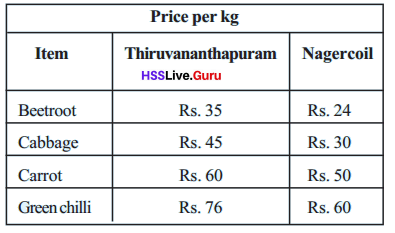Why are the prices different?

• Transportation

Majid is a vegetable merchant. He bought yam at 20 rupees a kilogram. He sold them on the spot at 25 rupees a kilogram. How much profit did he make in this sale?

• How many kilograms of yam did he buy?

Answer: He bought 1 kilograms of yam.

• How much money did he get on selling?

Answer: He got 25 rupees on selling.

• How much money did he spent on buying?

• How much is the profit?

Answer: He gained profit of 25-20=5 rupees.

The next day also, Majid bought 200 kilograms of yam at 20 rupees a kilogram and paid 200 rupees to take them to another market. There he sold them at 25 rupees a kilogram. How much is his profit?
Here, the vehicle fare also should be added to the amount paid for the yam to find the total amount he spent.
Given that
Majid bought 200 kilograms of yam at 20 rupees a kilogram and paid 200 rupees to take them to another market. There he sold them at 25 rupees a kilogram.
Step1: He bought 200 kilograms of yam at 20 rupees a kilogram, 200×20 rupees=4000 rupees and paid 200 rupees to take them to another market. So, the total money spent will be 4200 rupees.
Step 2:He sold 200 kilograms of yam at 25 rupees a kilogram which will be 5000 rupees. So, he gains a profit of 5000-4200=800 rupees.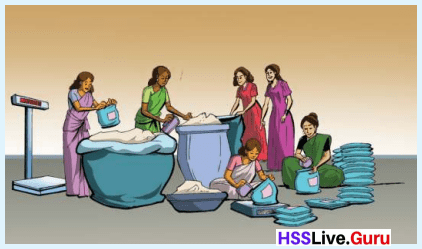At Oruma Cooperative Society, loo kilogram of wheat grains was bought at 25 rupees a kilogram. 500 rupees was spent in washing, drying, grinding and packaging. 100 packets were made, to be sold at 35 rupees a packet. 20 packets of powder was spoiled and the remaining sold. Did they make a profit or suffer a loss in this sale? How much?
Given that
Society bought 100 kilograms of wheat grains at 25 rupees a kilogram. 500 rupees was spent in washing, drying, grinding and packaging.100 packets were made, to be sold at 35 rupees a packet. 20 packets of powder was spoiled and rest were sold.
Step 1: Society bought 100 kilograms of wheat grains at 25 rupees a kilogram which will cost 2500 rupees and spent 500 rupees for washing, drying, grinding and packaging. So, the total cost price will be 3000 rupees.
Step 2: 100 packets were made, out of which 20 packets were spoiled. So, 80 packets were sold at 35 rupees a packet, 80×35=2800 rupees.
Step 3: As the selling price is lesser than the cost price, society will face a loss of 200 rupees.

• Thomas bought 10 cents of land at 75000 rupees a cent. He spent 50000 rupees to build a wall around and 60000 rupees to dig a well. He sold the plot at 90000 rupees a cent. Was it a gain or loss for him? How much?
Given that
Thomas bought 10 cents of land at 75000 rupees a cent. He spent 50000 rupees to build a wall around and 60000 rupees to dig a well.He sold the plot at 90000 rupees a cent.
Step 1: He bought 10 cents of land at 75000 rupees, 75000×10=750000 rupees.
Step 2: He spent 50000 rupees to build a wall and 60000 rupees to dig a well, so the total money spent will be 110000 rupees and the total price will be 750000+110000=860000 rupees.
Step 3: He sold the plot at 90000 rupees a cent, 90000×10=900000 rupees, which gains him a profit of 900000-860000=40000 rupees.

• A trader bought 20 quintals of rubber sheets at 19850 rupees a quintal. He spent 3000 rupees to take the load to the shop. Since prices went down, he had to sell them at 18250 rupees a quintal. How much money did he lose?
Given that
A trader bought 20 quintals of rubber sheets at 19850 rupees a quintal. He spent 3000 rupees to take the load to his shop. As prices went down, he sold them at 18250 rupees a quintal.
Step 1: He bought 20 quintals of rubber sheets at 19850 rupees a quintal, 20×19850=397000 rupees and spent 3000 rupees to take the load to the shop. So, the total amount spent will be 400000 rupees.
Step 2: He sold them at 18250 rupees a quintal, 20×18250=365000 rupees, which is less than the amount he spent.So his loss will be 400000-365000=35000 rupees.Selling fruit

This is the price list at Saji’s Fruit shop: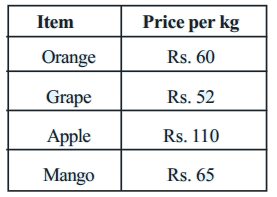He buys oranges and mangoes at 50 rupees a kilogram, grapes at 40 rupees a kilogram and apples at 100 rupees a kilogram. Which fruit gets him the most profit?

Oranges bought for 50 rupees are sold for 60 rupees and mango bought for 50 rupees are sold for 65 rupees. Of these, mangoes are more profitable, since they return more for the same amount spent.

When apples bought for 100 rupees are sold for 110 rupees he gets a profit of 10 rupees. When oranges bought for 50 rupees are sold for 60 rupees, how much profit does he get? How do we decide which is more profitable?
He gets the same profit of 10 rupees, on selling oranges bought for 50 rupees and apples bought for 100 rupees. Of these, oranges are more profitable, since he spends less on them.

Grapes bought for 40 rupees are sold for 52 rupees. Oranges bought for 50 rupees are sold for 60 rupees. Which of these is more profitable?
Let’s suppose he buys each for 100 rupees.
2 kilograms of oranges can be bought for 100 rupees and they are sold for 60 × 2 = 120 rupees; the profit is 20 rupees.
How many kilograms of grapes can he buy for 100 rupees? He can buy 2 kilograms for 80 rupees and with the remaining 20 rupees another $$\frac{1}{2}$$ kilogram; altogether 2$$\frac{1}{2}$$ kilograms.
For how much does he sells these?
52 × 2$$\frac{1}{2}$$ = 104 + 26 = Rs. 130
So the profit is 30 rupees.

Here we find that grapes fetch more profit, by assuming the amount spent on each to be 100 rupees.
Such computations can be made easier by reducing to percentages.
Let’s do these in percentages.

In selling oranges, the profit is $$\frac{10}{50}$$ = $$\frac{1}{5}$$ of the amount spent.
$$\frac{1}{5}$$ means $$\frac{1}{5}$$ × 100 = 20 percent.
In the case of grapes, the profit is $$\frac{12}{40}$$ = $$\frac{3}{10}$$ of the amount spent.
In terms of percentage, $$\frac{3}{10}$$ × 100 = 30%.
Similarly,
Profit for apples is $$\frac{10}{100}$$ × 100 = 10%
Profit for mangoes is $$\frac{15}{50}$$ × 100 = 30%
Thus the most profitable deals are in grapes and mangoes, each of these giving 30% profit.Let’s look at another problem:

• A man bought coconuts for 650 rupees and sold these for 598 rupees. What is the percent of loss?
The loss is 52 rupees.
It is $$\frac{52}{650}$$ = $$\frac{2}{25}$$ of the amount spent.
As a percent, $$\frac{52}{650}$$ × 100 = 8%

• An almirah bought for 5000 rupees was sold for 5600 rupees. What is the profit percent?
Given that
An Almirah is bought for 5000 rupees and was sold at 5600 rupees.
Step 1: Cost price of almirah is 5000 rupees.
Step 2: Selling price of almirah i s 5600 rupees.
Step 3: As selling price is greater than cost price, profit will be 5600-5000=600 rupees.
Step 4: In terms of percenatge,  $$\frac{600}{5000}$$×100 =12%

• A TV bought for 12000 rupees was sold for 10200 rupees. What is the loss percent?
Given that
A TV bought for 12000 rupees was sold for 10200 rupees.
Step 1: Cost price of TV is 12000 rupees.
Step 2: Selling price of TV is 10200 rupees.
Step 3: As selling price is lesser than the cost price, loss will be 12000-10200=1800 rupees.
Step 4, In terms of percentage, $$\frac{1800}{12000}$$×100=180%.

• Akhil is a fish vendor. One day he bought 12 kilograms of fish at 140 rupees a kilogram. He spent 120 rupees to take this to the shop. 4 kilograms of fish were spoilt and he sold the rest at 180 rupees a kilogram. Did he gain or lose money in this ? What percent?
Given that
Akhil is a fish vendor. One day he bought 12 kilograms of fish at 140 rupees a kilogram. He spent 120 rupees to take this to his shop. 4 kilograms of fish were spoilt and he sold the rest at 180 rupees a kilogram.
Step 1: He bought 12 kilograms of fish at 140 rupees, 12×140=1680 rupees and spent 120 rupees to take this to his shop. So, the total amount spent will be 1680 +120=1800 rupees.
Step 2: 4 kilograms of fish was spoilt. So, 8 kilograms of fish was sold at 180 rupees a kilogram, 8×180=1440 rupees.
Step 3: As cost price is greater than the selling price, he will face a loss of 1800-1440=360 rupees.
Step 4: In terms of percentage, $$\frac{360×100}{1800}$$=20%

• In Omega stores, Ceiling fans sold at 1728 rupees a piece fetches 128 rupees as profit, while pedestral fans sold at 2616 rupees a piece fetches 216 rupees as profit. Which sale is more profitable?
Given that
Ceiling fans are sold at 1728 rupees a piece fetches 128 rupees as profit, while pedestral fans sold at 2616 rupees a piece fetches 216 rupees as profit.
Step 1: Ceiling fans sold at 1728 rupees a piece and fetches profit of 128 rupees, so the cost price will be 1728-128=1600 rupees.
Step 2: In terms of percentage, $$\frac{128}{1600}$$×100=8%.
Step 3: Pedestral fans sold at 2616 rupees a piece and fetches profit of 216 rupees, so the cost price will be 2616-216=2400 rupees.
Step 4: In terms of percentage, $$\frac{216}{2400}$$×100 = 9%.
So, pedestral fans sale is more profitable.

• A retailer buys 150 kilograms of pepper at 400 rupees a kilogram and sells it at a profit of 60 rupees a kilogram.

• What is the total amount spent in buying?
Let us assume that X is weight of pepper and Y is the price paid for 1 kilogram.
The total amount spent in buying 150 kilograms will be 150×400 = 60000 rupees.

• What is the total amount got?
Let us assume P as profit per kilogram.
The total amount he got is 150× (400+60) = 69000 rupees.

• What is the total profit?
Pepper bought for 60000 are sold at 69000, which gains profit of 69000-60000=9000 rupees.

• What is the profit percent?
In terms of percentage, $$\frac{9000}{60000}$$×100 = 15%.

Some other sums Textbook Page No. 134

A trader bought an electric iron for 1200 rupees. He wants 12% profit on selling it. At what price should he sell it?
Here, the iron is bought for 1200 rupees.
And the profit wanted is 12% of this.
That is, 1200 × $$\frac{12}{100}$$ = 144 rupees.
Now to find the sale price, we need only add the profit to 1200 rupees.
We can also directly compute 112% of 1200 rupees.
1200 × $$\frac{112}{100}$$ = 1344 rupees
If a sale results in 10% loss, what percent of the investment is the sale price?
Calculate the sale price of each in the table below: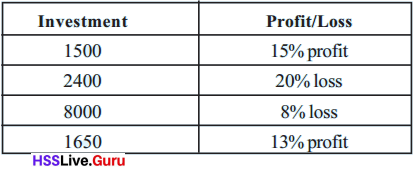Case 1:
15% of profit is achieved for 1500 rupees investment.
Therefore, the sale price will be $$\frac{(100+15)}{100}$$×1500=1725 rupees.
Case 2:
20% loss is observed for 2400 rupees investment.
Therefore, the sale price will be $$\frac{(100-20)}{100}$$×2400=1920 rupees.
Case 3:
8% loss is observed for 8000 rupees investment.
Therefore, the sale price will be $$\frac{(100-8)}{100}$$×8000=7360 rupees.
Case 4:
13% of profit is achieved for 1650 rupees investment.
Therefore, the sale price will be $$\frac{(100+13)}{100}$$×1650= 1864.5 rupees.

A trader sold a bicycle for 4500 rupees, at 10% loss. What amount did he originally spent on it?
Since the loss is 10%, the sale price is 90% of the original investment. Thus
Original investment × $$\frac{90}{100}$$ = 4500
From this, we can compute the original investment as 10
4500 × $$\frac{10}{9}$$ = 5000 rupees.

• Compute the investment: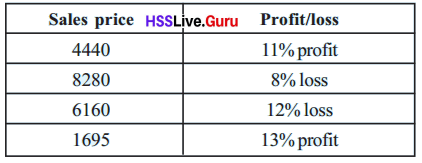• 10 kilogram of tomatoes were bought for 270 rupees. Of this, one kilogram was spoilt. To get 20% profit, at what price a kilogram should the remaining be sold?
Given that
10 kilogram of tomatoes were bought for 270 rupees. Of this, one kilogram was spoilt.
Step 1: 10 kilogram of tomatoes were bought for 270 rupees. Let us make an assumption that 100% cost of tomatoes is 270 rupees.
Step 2: To get 20% more profit, $$\frac{270×120}{100}$$ =324 rupees.
Step 3: If 1 kilogram of tomato is spoiled than 9 kilogram of tomatoes should be sold at 324 rupees. So, the selling price for 1 kilogram of tomato will be $$\frac{324}{9}$$=36 rupees.
To get 20% profit, tomatoes should be sold at 36 rupees per kilogram.

• Shine sold two tables at 9900 rupees each. He got 10% profit in one sale and 10% loss in the other. Did the total sale result in a profit or loss? What percentage?
Given that
Shine sold two tables at 9900 rupees each. He got 10% profit in one sale and 10% loss in the other.
Step 1: He sold first table at 9900 rupees and got profit of 10%.  So the cost price of the table will be $$\frac{100}{110}$$×9900 = 9000 rupees.
Step 2: He sold second table at 9900 rupees with 10% loss. So the cost price of the table will be $$\frac{100}{90}$$×9900=11000 rupees.
Step 3: Total selling price of both tables will be 9900+9900=19800 and total cost price will be 9000+11000 = 20000 rupees.As cost price is greater than selling price, he will face loss of 20000-19800=200 rupees.
Step 4: In terms of percentage, $$\frac{200}{20000}$$×100 = 1%

• A trader makes 20% profit in selling a washing machine at 12000 rupees. How much would he have in vested in the machine? During new year sales, its price in reduced by 1200 rupees. Is the sale now at a profit or loss? What is the percent?
Given that
A trader makes 20% profit in selling a washing machine at 12000 rupees.
Step 1: A trader is selling washing machine at 12000 rupees and makes 20% profit. So, the cost price of the washing machine will be $$\frac{12000}{120}$$×100 =10000 rupees.
Step 2: During new year sales, the price reduced by 1200 rupees. So, the new selling price will be 12000-1200=10800 rupees.
Step 3: As the new selling price is greater than the cost price, he earns a  profit of 10800-10000=800 rupees.
Step 4: In terms of percentage, $$\frac{80}{10000}$$×100 =8%Discount

Haven’t you seen such ads during festive seasons?Many shops try to increase sales by reducing the prices. Such a decrease in price is called discount.

For example in a shop, a shirt normally sold for Rs. 500 is given 20% discount means the customers can get it for
20% less than 500 rupees; that is
500 × $$\frac{80}{100}$$ = 400 rupees

Here, the price shown on the shirt in called the regular price. The discount is often given as a percent of the regular price.

• George bought an almirah at 8% discount. The price reduction was 960 rupees. What is the regular price? How much did George actually pay for it?
Discount is 5% of the regular price
So, regular price × $$\frac{8}{100}$$ = 960 rupees
From this, the regular price can be calculated as
960 × $$\frac{100}{8}$$ = 12000 rupees.

Subtracting the discount amount from this gives the amount George paid.
Regular price of the almirah is 12000 rupees and discount price is 960 rupees.
So, the amount he paid will be 12000-960 = 11040 rupees.

• The price of a gold sovereign (8 grams) is 22500 rupees. For jewellery, manufacturing charge is 6% of the price of gold. A shop offers 20% discount on this charge. How much money is needed to buy a bangle of one sovereign from here?
Manufacturing charge is 6% of the price of gold.
Manufacturing charge = 22500 × $$\frac{6}{100}$$
= 1350 rupees
Since 20% discount is given on this, only 80% of 1350 need be paid. So manufacturing charge after discount is
1350 × $$\frac{80}{100}$$

Now to find the price of the bangle, we add this to the price of gold.
Manufacturing charges after discount will be 1080 rupees.
Now, the price of the gold will be 22500+1080=23580 rupees.

• A person bought khadi clothes on Gandhi Jayanthi day, when 30% rebate was given. He paid 3500 rupees. What is the regular price of clothes he bought? The reduction is 30%. So he paid only 70%.
That is, regular price × $$\frac{70}{100}$$ = 3500
From this can’t you find the regular price?
Regular price will be $$\frac{3500×100}{70}$$=5000 rupees.

• Look at two ads in a TV shop: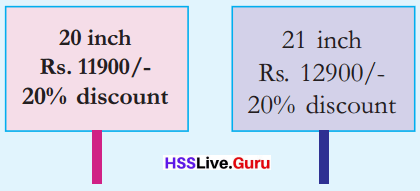• If one has only 10000 rupees with him, which of these TV’s can he buy?
Given that
A TV shop is selling 20 inch TV at 11900 rupees with 20% discount and 21 inch TV at 12900 rupees with 20% discount.
Step 1: The discount price in first case will be 20% of regular price, $$\frac{20×11900}{100}$$ = 2380 rupees.
So, the selling price of the TV will be 11900-2380 = 9520 rupees.
Step 2: The discount price in second case will be 20% of regular price, $$\frac{20×12900}{100}$$ = 2580 rupees.
So, the selling price of the TV will be 12900-2580=10320 rupees.
Therefore, if a person has 10000 rupees, he can buy 1st TV as its selling price will be 9520 rupees.

• What is the difference in prices after discount?
Cost of first TV after discount will be 9520 rupees.
Cost of second TV after discount will be 10320 rupees.
Difference in prices after discount will be 800 rupees.

• In a furniture shop, a cot and an almirah sold separately for Rs. 15000 and Rs. 25000, are sold together for Rs. 36000. What is the discount percent in this deal?
Given that
In a furniture shop, a cot and an almirah sold separately for Rs. 15000 and Rs. 25000, are sold together for Rs. 36000.
Step 1: The selling price of the cot is Rs.15000 and almirah is Rs. 25000. So, the total  selling price of both the products will be Rs. 40000.
Step 2: Selling price of the products sold altogether is Rs.36000. So, the discount price will be 40000-36000=Rs.4000.
Step 3: In terms of percentage, $$\frac{4000×100}{40000}$$= 10%
Therefore, the deal will have 10% discount.

• Susan and Gayatri bought copies of an English-Malayalam dictionary each for 490 rupees. To get 20% discount, they decided to pay the bill together. When the salesman told them they would get 30% discount on purchase above 1000 rupees, each bought a picture book for 60 rupees and paid the bill together.• How much did they pay together? What is the share of each?
Given that
Susan and Gayatri bought copies of an English-Malayalam dictionary each for 490 rupees. To get 20% discount, they decided to pay the bill together. Each bought a picture book for 60 rupees.
Step 1: The marked price for each dictionary is 490 rupees. So, the total amount spent on dictionary will be 980 rupees.
Step 2: The marked price for each picture book is 60 rupees. So, the total amount spent on picture book will be 120 rupees.The intotal amount spent on both books will be 980+120=1100 rupees.
Step 3: 30% of discount is applicable on purchase of above 1000 rupees. So, the discount price will be $$\frac{30}{100}$$ ×1100=330 rupees.
Total amount to be paid by them will be 1100-330= 770 rupees.

• If both had bought only the dictionaries and paid the bill together, how much would they have paid? What would be the share of each?
Step 1: The total amount spent on dictionaries by both is 980 rupees.
Step 2: 20% of discount is applicable on purchase of amount in between 500 and 1000 rupees. So, the discount price will be $$\frac{20}{100}$$×980 = 196 rupees.
Total amount they would have paid will be 980-196=784 rupees.

• If each had bought these two books separately, how much would each have spent?
Step 1: Amount spent by sushan for both books would be 490+60=550 rupees. 20% of discount is applicable on purchase of amount inbetween 500 and 1000 rupees. So, the discount price will be $$\frac{20×550}{100}$$=110 rupees.
The amount paid by Sushan will be 550-110=440 rupees.
Step 2: Amount spent by Gayatri for both books would be 490+60=550 rupees. 20% of discount is applicable on purchase of amount inbetween 500 and 1000 rupees. So, the discount price will be $$\frac{20×550}{100}$$ = 110 rupees.
The amount paid by Gayatri will be 550-110=440 rupees.

• The bill below shows cloths bought from a khadi outlet. What is the total amount to be paid?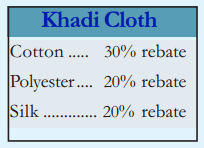Given that
The shopkeeper proovides 30% of rebate on cotton, 20% of rebate on polyester and 20% of rebate on silk. The total amount paid will be Rs. 2190.
Step 1: Amount spent on cotton clothes will be 350+550=Rs. 900. Regular price after 30% of rebate on cotton will be $$\frac{900×30}{100}$$= Rs.270. The amount paid for cotton dhoti and shirt will be 900-270=Rs.630.
Step 2: Regular price after 20% of rebate on polyester will be $$\frac{450×20}{100}$$=Rs.90. The amount paid for polyester shirt will be 450-90=Rs.360.
Step 3: Regular price after 20% of rebate on silk will be $$\frac{1500×20}{100}$$=Rs. 300. The amount paid for silk saree will be 1500-300=Rs.1200.
Therefore, the total amount paid will be 630+360+1200 = Rs. 2190

• A trader buys an electric fan for Rs. 2500. He raises the price by 40% and then offers 15% discount. At what price does he sells it?
Given that
A trader buys electric fan for Rs. 2500. He raises the price by 40% and then offers 15% discount.
Step 1: He bought the electric fan for Rs. 2500.
Step 2: Price after raising by 40% will be $$\frac{40×2500}{100}$$=Rs.1000. So, the total price will be Rs. 3500.
Step 3: After applying 15% of discount, the discount price will be $$\frac{15×3500}{100}$$=Rs.525. So, the selling price will be 3500-525=Rs. 2975
Hence, he should sell the fan at Rs.2975.

• A gas stove is bought for Rs. 3600. What should be the price marked on it, if 20% profit is to be got, after 10% discount.
Given that
A gas stove is bought for Rs.3600. The marked price should be Rs.4800 to get 20% of profit after 10% of discount.
Step 1: A gas stove is bought for Rs.3600. Price after gaining the profit of 20% will be $$\frac{20×3600}{100}$$=Rs.720. So, the total selling price will be 3600+720=Rs. 4320.
Step 2: Let us assume that marked price is 100%. So, price after 10% discount will be 90%
$$\frac{4320×100}{90}$$ =Rs.4800

• A shop offers an electric iron free with a fridge. They sell the fridge for Rs. 9000 and the iron for Rs. 1000. If 20% profit is to be got on this deal, what should be the selling price of the fridge?
Given that
A shop sells fridge for Rs.9000 and the iron for Rs.1000. Inorder to get 20% profit on the product, the selling price should be Rs.12000.
Step 1: A shop sells fridge for Rs.9000 and iron for Rs.1000. So, the total price will be Rs.10000.
Step 2: Price to get 20% profit will be $$\frac{10000×20}{100}$$=Rs.2000. So, the total selling price should be 10000+2000=Rs.12000

Interest Textbook Page No. 140

Have you seen ads like these in front of banks?
We approach banks for depositing money and getting loans.Amal deposited 15000 rupees in a bank. After one year, he got 16500 rupees back. How much more did he get?
This extra money is called interest. What if we take out a loan from a bank?
Given that
Amal deposited 15000 rupees in a bank. After one year, he got 16500 rupees back.He received interest of 1500 rupees.
Step 1: Amal deposited 15000 rupees and got 16500 rupees after one year.
So, the extra amount he received is 16500-15000=1500 rupees.Interest rate

Thomas took out 50000 rupees as loan from a bank. After one year, he had to pay back 52000 rupees.
How much is the interest?
What percent of the loan amount is this?
$$\frac{2000}{50000}$$ × 100 = 4%
For one year, he paid back 4% more than the loan.
It is called the rate of interest
If 1500 rupees is the interest got in a year for a deposit of 15000 rupees, then the rate of interest is,
$$\frac{1500}{15000}$$ × 100 = 10%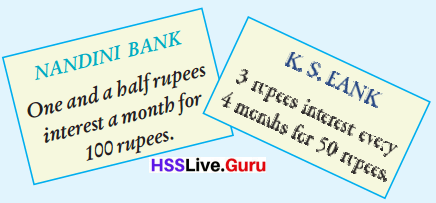Which bank gives more interest?
Let’s look at Nandini Bank.
One month’s interest for 100 rupees is 1$$\frac{1}{2}$$ rupees.
One year’s interest for 100 rupees 12 × 1$$\frac{1}{2}$$ = 18 rupees.
So, the rate of interest is 18%
4 month’s interest for 50 rupees is 3 rupees.
4 month’s interest for 100 rupees is 3 × 2 = 6 rupees.
One year’s interest for 100 rupees is 6 × 3 = 18 rupees.
So in this bank also, the rate of interest is 18%.

Compute the rate of interest in each case:Case 1:
Interest for Rs.500 for 1 year is Rs.30.
So, the rate of interest will be $$\frac{30×100}{500×1}$$ = 6%
Case 2:
Interest for Rs.1000 for 4 months or 1/3 year is Rs.40.
So, the rate of interest will be $$\frac{40×100}{1000×4/12}$$ = 12%
Case 3:
Interest for Rs.200 for 2 months or 1/6 year is Rs.2.
So, the rate of interest will be $$\frac{2×100}{200×2/12}$$ = 6%
Case 4:
Interest for Rs.2 for 1 month or 1/12 year is Rs.3
So, the rate of interest will be $$\frac{3×100}{2×2/12}$$ = 1800%
Case 5:
Interest for Rs.5000 for 2 years is Rs.1200.
So, the rate of interest will be $$\frac{1200×100}{5000×2}$$ =12%

As time changes

The Co-operative Bank gives 9% interest for fixed deposits. Ravi deposited 30000 rupees. How much would he get after a year?
The interest for one year is 9% of the deposit.
That is,
30000 × $$\frac{9}{100}$$ = 2700 rupees
So he gets 32700 rupees back.
Suppose he withdraws the amount only after two years.

He gets interest for two years; that is,
2 × 2700 = 5400 rupees We can compute two years interest directly as,
30000 × $$\frac{9}{100}$$ × 2 = 5400 rupees
What about interest for three years?
Interest for three years can be calculated as  $$\frac{30000×3×9}{100}$$ =8100 rupees.
Similarly, What is the interest for 2000 rupees at 8% for 4 years?
Interest for 2000 rupees at 8% for 4 years will be  $$\frac{2000×4×8}{100}$$ =640 rupees.

• Suma deposited 25000 rupees in a bank, which gives 11% interest. How much would she get back after 3 years?
We can directly compute the interest for 3 years.
25000 × $$\frac{11}{100}$$ × 3 = 8250 rupees
To find the amount she would get back, we need only add this interest to the deposit.
How much is it?
Given that
Suma got 8250 rupees as interest amount for 25000 rupees deposit at 11% interest for 3 years.
Total amount she will get after 3 years is 25000+8250=33250 rupees.

• Vijayan took out a loan of 50000 rupees at 12% interest. After two years, he paid back 25000 rupees. How much should he pay back after one more year?
Here some money is paid back after two years.
So let’s first calculate the interest for two years.
50000 × $$\frac{12}{100}$$ × 2 = 12000 rupees
The actual amount to be paid after two years
50000 + 12000 = 62000 rupees
Of this 25000 rupees was given. Remaining amount is
62000 – 25000 = 37000 rupees
So the amount to be paid back after one more year is 37000 rupees and the interest on it. Can’t you compute it?
Interest amount for 37000 rupees after 1 year at 12% interest will be $$\frac{37000×1×12}{100}$$  = 4440 rupees.
Therefore, the amount to be paid after one more year will be 37000+4440=41440 rupees.

• Babu deposited 25000 rupees in a bank, which gives 15% interest. How much would he get back after two years?
Given that
Babu deposited 25000 rupees in a bank, which gives 15% intrerest.
Step 1: He deposited 25000 rupees at 15% interest. So, the interest amount will be $$\frac{25000×2×15}{100}$$ = Rs.7500
Step 2: Amount he will be getting back after 2 years will be 25000+7500=32500 rupees.

• Dileep took out a loan of 36000 rupees from a bank, which charges 10% interest. He decided to pay back the total amount including interest for 2 years, in 24 monthly installments. How much should he pay every month?
Given that
Dileep took out loan of 36000 rupees from a bank at 10% interest. He decided to pay back the total amount including interest for 2 years.
Step 1: He took 36000 rupees at 10% interest for 2 years. So, the intrest amount will be $$\frac{36000×2×10}{100}$$ =7200 rupees.
Step 2: Amount he should pay for 2 years will be 36000+7200=43200 rupees. His monthly installements will be $$\frac{43200}{24}$$ =1800 rupees.

• Johny deposited 60000 rupees in a bank, which gives 1 paise interest on one rupee every month. How much would he get back after two years?
Given that
Johny deposited 60000 rupees in a bank at 1 paise interest on one rupee every month.
Step 1: Johny deposited 60000 rupees. Interest for the one month will be $$\frac{1×60000}{100}$$=600 rupees.
Interest amount for 2 years will be 24×600=14400 rupees.
Step 2: Amount he will be getting after 2 years will be 60000+14400=74400 rupees.

• Sujith and Anish took out agricultural loans, 50000 rupees each from a bank at 4% interest. Suj ith settled the debt after one year and took out another loan of 50000 rupees, which he settled the next year. Anish could not settle after one year and he was asked to pay 7% interest for the second year. How much did each give as interest?
Given that
Sujith and Anish took loan of 50000 rupees at 4% interest. After 1 year sujith settled the loan and took other loan. Anish could not settle and he paid 7% interest for second year.
Step 1: Sujith took 50000 rupees loan at 4% of interest for 1 year as he settled loan in first year. So, the amount paid for a year will be $$\frac{50000×1×4}{100}$$ =2000 rupees.
Sujith took another loan of 50000 in second year, so he will again pay Rs. 2000 as interest amount.
Total amount he paid in two years will be 2000+2000=4000 rupees.
Step 2: Anish took 50000 rupees loan at 4% of interest. Amount he will pay in first year will be $$\frac{50000×1×4}{100}$$ =2000 rupees.
In second year, his interest rate was 7%. So, the amount he will pay in second year will be $$\frac{50000×1×7}{100}$$ = Rs. 3500.
Total interest amount he paid in two years will be 2000+3500=5500 rupees.

• Rahul and Raj ani opened accounts in a bank on the same day, each depositing 8000 rupees. After one year, Rahul withdrew the entire amount including interest and re-deposited it. After one more year, both closed their accounts. How much did each get? Why are the amounts different?
Step 1: Rahul deposited 8000 rupees in the first year. Let us assume 10% as interest rate. So, the interest for first year will be $$\frac{8000×1×10}{100}$$ =800 rupees.
Step 2: He re-deposited 8800 rupees at 10% interest rate for one more year. So, the interest amount for second year will be $$\frac{8800×1×10}{100}$$=880 rupees.
Step 3: Rajani deposited 8000 rupees for 2 years.Let us assume the same the interest rate as above. So, the interest for 2 years will be $$\frac{8000×2×10}{100}$$=1600 rupees.